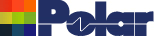Asymmetric coplanar and differential coplanar controlled impedance structures Model More Structures: Two tricks that enable you to model structures that aren’t included in the Si8000m and Si9000e No matter how many structures we include in the Si8000m and Si9000e it seems that there are never enough. If we don’t have the particular structure that you need, here are two tricks that may help you to work around the problem and calculate good, accurate values. Increase structure dimensions One example of this technique is to model a coplanar structure that has only half a ground plane. We have encountered this semi-coplanar structure (sometimes referred as uniplanar) in certain proprietary applications, particularly for consumer electronics. A semi-coplanar or asymmetric coplanar structure may look like Figure 1.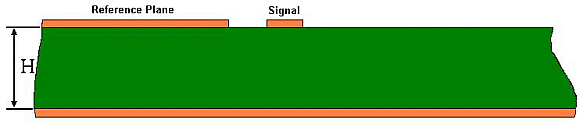Figure 1 The Si8000m does not have a structure exactly like this, but it does have the Differential Surface Coplanar Waveguide structure below in Figure 2 which is similar.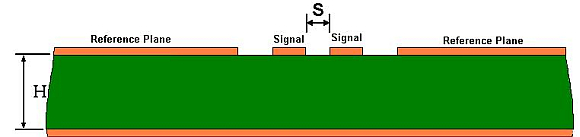Figure 2 By increasing the distance between the differential signal tracks, S, to a large number such that the impedance no longer increases with any further increase in S, we can emulate figure 1. For typical structure dimensions, increasing S to 1000 times H, the dielectric thickness, will be adequate. This arrangement is shown in Figure 3.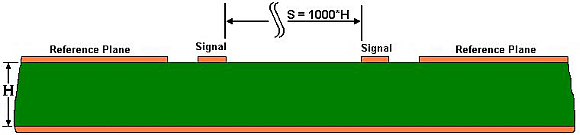Figure 3 At this distance apart, the two (differential) signal tracks are no longer coupled, but the Si8000m field solver still accurately calculates Zo(diff) for the structure. Remember that Zo(diff) for the differential pair is equal to twice Zo(odd) for a single track which will be the characteristic impedance of this single ended track. An example of this calculation using the Si8000m Quick Solver is shown below in figure 4.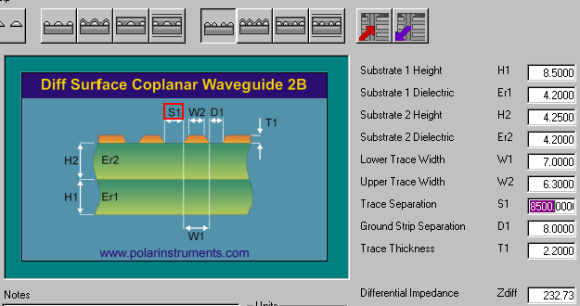Figure 4 Zo(diff) is calculated to be 244 ohms, so dividing by two yields a value of 122 ohms for the semi-coplanar structure. Another example of using large distances to separate out unwanted components might be to calculate the impedance of a differential pair that exists in the absence of a reference plane. Although such a structure is theoretically unsatisfactory, it is sometimes used as a compromise to achieve cost economies. To model it, we may use the Si8000m Differential Surface Coplanar Waveguide by spacing the coplanar reference planes far away from the signal tracks until there is no further increase in impedance. This time, the Si8000m Excel layout has been used to illustrate the effect in figure 5, below.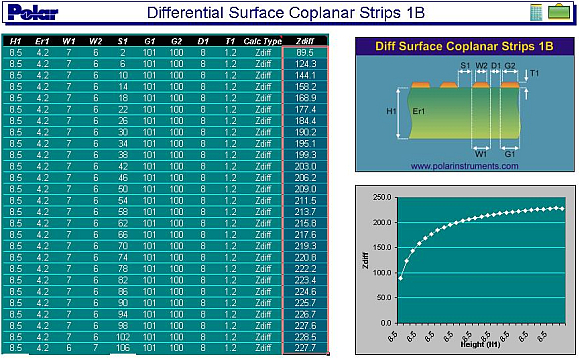Figure 5 Notice how increasing S beyond 74 mils (60 times the copper thickness in the case of this structure) achieves virtually no further increase in impedance. The impedance of the an asymmetric coplanar structure in the absence of reference tracks or planes will be 112 ohms for these dimensions. Use virtual grounds We often don’t realise that a virtual ground exists between conductors driven differentially (driven in odd mode.) Consider the conductors in figure 6 when they are driven differentially.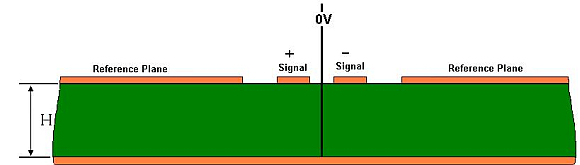Figure 6 Since the tracks are driven in equal but opposite sense, the electric field at every point equidistant from the two is at 0V (half way between +V and –V). We can use this to our advantage to simplify some calculations, or to model certain structures. Consider the customer’s structure that was presented to Polar in Figure 7, below.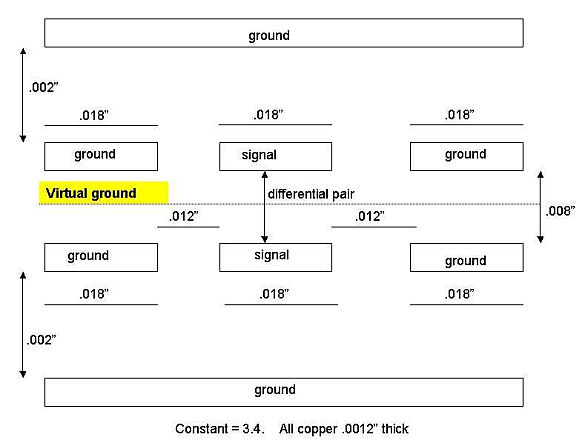Figure 7 The Si8000m does not contain a structure identical to this, but it does have the Offset Coplanar Strips model, shown in figure 8, below.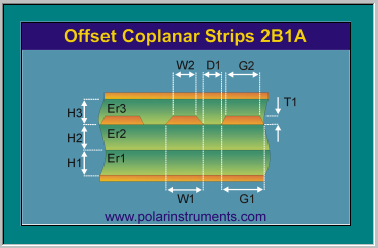Figure 8 It can easily be seen that this structure is one half of the structure in figure 7. The lower planes correspond, the signal and ground (reference) tracks in figure 8 represent the lower signal and ground tracks in figure 7, and the upper reference plane in figure 8 corresponds to the virtual ground plane in figure 7. A similar correspondence can be seen for the upper half of figure 7. For the purpose of this technique, we consider that in figure 8, only one signal track is driven with voltage V. In figure 7, both signal tracks are driven, one by +V and the other by –V. So in figure 7, the driving voltage is double that in figure 8 ( = +V–(–V) = 2V), but the current will be the same. Therefore the impedance in figure 7 is double that in figure 8. Once again, by using figure 8 to determine the Zo(odd) for one of the differential tracks in figure 7, we can determine the Zo(diff) for the pair by using the relationship Zo(diff) = 2*Zo(odd). Once again, the Quick Solver is conveniently used for the purpose, as shown in figure 9.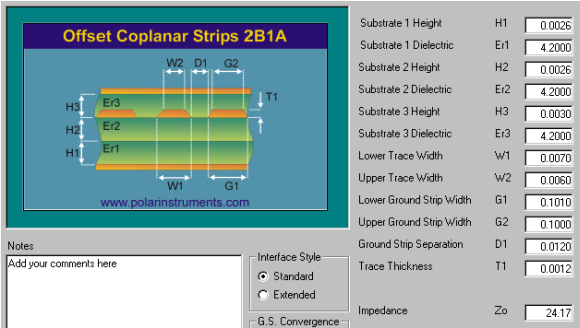Figure 9 Zo(odd) is calculated to be 24.17 ohms, therefore Zo(diff) for the compound structure in figure 7 is 2 * 24.17 = 48.34 ohms. Conclusions The structures provided in Si8000m may be looked at as tools. Look beyond the immediate purpose of the tools to see if they can be adapted to suit your different and individual needs. Particularly useful techniques are to increase distances to remove the effect of some structural elements, and to look for virtual ground planes between differential tracks to see if a single ended model can be adapted to fit your needs. Note also how the Excel table and chart conveys a helpful and illuminating impression of what is happening to impedance as the dimensions vary. Note the flexibility of the Quick Solver in adapting to the different measurement units. It can even accept mixed units for a calculation; for example for a single calculation some dimensions may be entered in mils, the rest in microns.